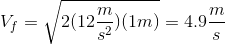# MCAT Physical : Pendulums

## Example Questions

### Example Question #1 : Pendulums

Which of the following changes would increase a pendulum's frequency?

Increasing the mass at the end of the pendulum

Decreasing the angle of displacement

Increasing the angle of displacement

Increasing the length of the pendulum's string

Shortening the length of the pendulum's string

Shortening the length of the pendulum's string

Explanation:

The only two factors that affect a pendulum's frequency are the acceleration due to gravity (g) and the length of the pendulum's string (L). This can be seen in the following formula: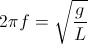### Example Question #1 : Pendulums

Which factors increase the maximum velocity of a pendulum?

Decreasing both the length of the pendulum's string and the angle of displacement

Decreasing the length of the pendulum's string and increasing the angle of displacement

Increasing both the length of the pendulum's string and the angle of displacement

Increasing the length of the pendulum's string and decreasing the angle of displacement

Increasing both the length of the pendulum's string and the angle of displacement

Explanation:

Both the length of the pendulum's string and the angle of displacement affect the maximum velocity of the pendulum. Increasing the length of the pendulum's string and increasing the angle of displacement both increase the distance the pendulum must travel in a single period, increasing its potential energy at its maximum height, and therefore the maximum velocity at its lowest point.

### Example Question #1 : Pendulums

What is the period of a pendulum that has a string length of 9.8m?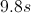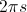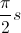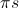Explanation:

The key to answering this question is to recall the following important formula for a simple pendulum: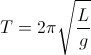.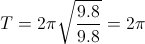### Example Question #2 : Pendulums

Which of the following changes to a pendulum will affect the angular velocity?

Raising the height from which the mass is released

Lowering the height from which the mass is released

Decreasing the length of the string

Increasing the mass on the end of the string

Decreasing the length of the string

Explanation:

For a pendulum, the angular velocity is given by the equation, where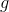is the acceleration due to gravity andis the length of the pendulum. Of the available answer choices, only changing the length of the string will effect the angular velocity.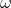does not depend on mass or the release point of the pendulum.

### Example Question #2 : Pendulums

The frequency of a pendulum on Earth is measured to be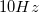. What will be the approximate frequency of the same pendulum on the moon?

Approximate gravity on moon is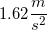.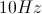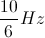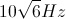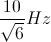Explanation:

The frequency of a pendulum is given by the relation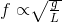.

Since gravity on the moon is one sixth of the gravity on Earth, frequency on the moon will be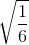that on earth.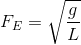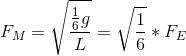If the frequency on Earth is, then the frequency of this pendulum on the moon is.

### Example Question #6 : Pendulums

The period of a certain pendulum is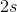. What is the period after doubling the mass at the end of the pendulum?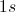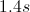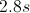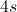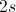Explanation:

The period of a pendulum is given by the formula: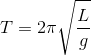is the length of the pendulum arm or string, andis the acceleration due to gravity. Gravity is constant, suggesting that the only way to manipulate the period of the pendulum is by adjusting the length of the string. Doubling the length of the pendulum arm would therefore increase the period. Since mass does not appear in the equation, it has no effect on the period of a pendulum.

### Example Question #1 : Pendulums

A pendulum is set in motion. Which of these statements correctly characterizes the mechanical energy in the system?

The mechanical energy varies from zero at the mid-point of the arc, to a maximum at the point of release

Ignoring friction, the mechanical energy is constant

The mechanical energy varies from zero at the point of release, to a maximum at the mid-point of the arc

The mechanical energy in the system varies from a maximum at the point of release to half the value of the maximum at the mid-point of the arc

None of these statements are true

Ignoring friction, the mechanical energy is constant

Explanation:

Mechanical energy is the sum of potential energy and kinetic energy. Mechanical energy is conserved, unless there is intervention by a non-conservative force (such as friction).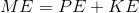The total energy in the system is determined by how far the pendulum is raised before release, based on the initial potential energy.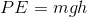The potential energy is all converted to kinetic energy as the pendulum swings through the bottom, or mid-point of its excursion.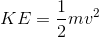Once the pendulum is set in motion, the mechanical energy is constant in a frictionless system.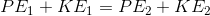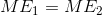### Example Question #1 : Pendulums

For a pendulum undergoing simple harmonic motion, the ratio of the weight of the pendulum and the displacement of the pendulum from the bottommost point in its path always equals __________.

the gravitational constant

the spring constant

the tension in the string

the sum of the kinetic and potential energy of the object

the spring constant

Explanation:

Hooke’s law, which is applicable to simple harmonic motion, states the relationship between force (F) and displacement (d).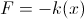k is equal to the spring constant. The ratio of F (force) to x (displacement) will be equal to the magnitude of k. In our set-up, the force is equal to the weight of the pendulum, so the ratio of weight to displacement is equal to the spring constant. This is true of all pendulums, and is given by the equation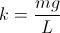.

### Example Question #9 : Pendulums

A pendulum has a length 2 meters with a 5kg mass at the end. It has a maximum angle of 60 degrees from vertical and is hanging from the ceiling of an elevator. When the pendulum is at its highest point, the elevator begins to accelerate at a rate of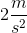. What is the the pendulum's new maximum velocity (neglecting the vertical velocity of the elevator)?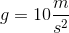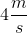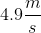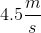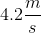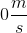Explanation:

This problem covers conservation of energy in the form of a pendulum: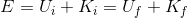If the initial state is when the pendulum is at its highest point, and the final state is when the pendulum is at its lowest state, we can rewrite: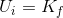Substituting in our expressions: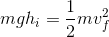Rearranging for final velocity: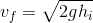We can calculate the height using the maximum angle the pendulum makes to the vertical. At this point, the pendulum covers a vertical distance of: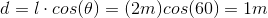Therefore, the height above the lowest point is: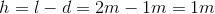Now, we just need to find out what the net downward acceleration is. Normally gravity is 10, but the elevator is accelerating upward at a rate of. Therefore the percieved gravitational acceleration is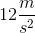.

Plugging in all of our values: